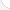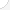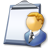Teachers-postsecondaryDescription: Postsecondary teachers work at colleges, community colleges, universities, and research facilities. They specialize in one field, such as history, physics, or journalism. They do research and write articles and books about their findings. Most professors hold advanced degrees. While many schools do not hold classes during the summer months, faculty work year-round, preparing lectures, attending seminars, and conducting research.Source: Bureau of Labor Statistics, U.S. Department of Labor, Occupational Outlook Handbook, 2008-09 Edition at http://www.bls.gov/OCO/Complete Job Profile: http://www.bls.gov/oco/ocos066.htmSalary: \$28,001 to \$50,000 per yearComments:I am a University Professor teaching Thermodynamics in Turin - paolog@polito.it (Paolo GREGORIO)

There are 46 math topics Teachers-postsecondary need to know.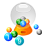Basic Math / Algebra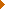FractionsDecimalsMetric MeasurementMeasurement ConversionPowers and RootsOther Number BasesNegative NumbersScientific NotationBasic Problem Solving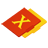First-Year AlgebraUsing FormulasLinear EquationsLinear InequalitiesOperations with PolynomialsFactoring PolynomialsRational ExpressionsCoordinate Graphing 2DLinear SystemsRadicalsQuadratic EquationsAlgebraic Representation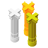Second-Year Algebra / TrigonometryFunctionsVariationImaginary NumbersPolynomial EquationsLogarithmsSequences and SeriesMatricesCoordinate Graphing 3DNon-Linear SystemsTrigonometric/Circular FunctionsGraphs of Trigonometric FunctionsTrigonometric IdentitiesTrigonometric Equations/InversesOblique TrianglesPolar Coordinates/GraphsVectors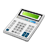Other TopicsCalculus and Higher MathBasic Calculator UseScientific Calculator UseComputer UseComputer ProgrammingGroup Problem SolvingMental MathInductive/Deductive ReasoningMath CommunicationsMathematical Modeling